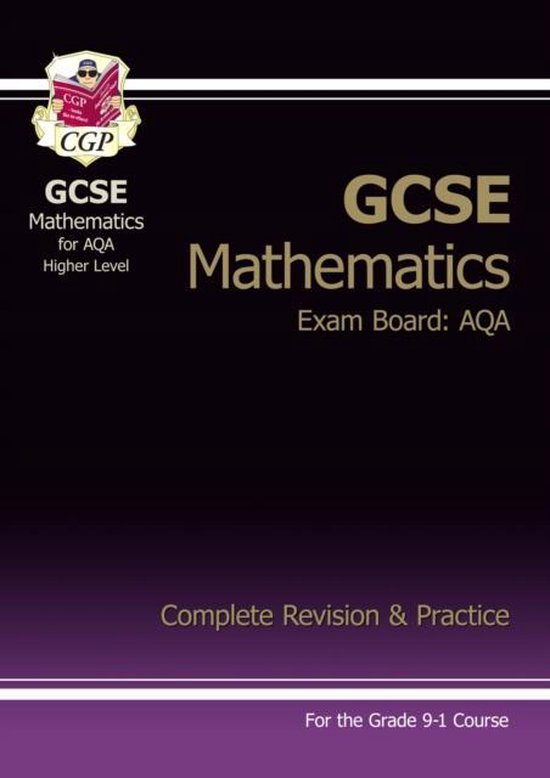# AQA · gcse maths · 9 1

Here are the best resources to pass 9 1 at AQA. Find 9 1 study guides, notes, assignments, and much more. We also have lots of notes, study guides, and study notes available for gcse maths at AQA.

### All 13 resultsAQA GCSE Maths - geometry & measures flashcards
(0)
\$4.10

These flashcards are useful in learning and memorising key topics within geometry & measures (across all exam boards), with diagrams to support your understanding. (topics included: angles in parallel lines, angles in polygons, special quadrilaterals)

•  Book
• Summary
•  • 5 pages •
• by justjosheela •
Quick View
i x
•
•
AQA GCSE Maths - notes on averages from tables
(0)
Free

This free document shows how to calculate averages (mode, median, mean) from tables through a worked example.

• Summary
•  • 1 pages •
• by justjosheela •
Quick View
i x
•#### Sell your knowledge on Stuvia

Hundred thousands of people are searching for your content every day. You can easily upload your summaries to our platform and start earning money from your study notes.AQA GCSE Maths - ratio & proportion flashcards
(0)
\$5.00

These flashcards are useful in learning and memorising key topics within ratio & proportion: each one shows the formula (both linear & triangles), with worked exam style examples. (topics included: indices & index laws, density, speed/distance/time, percentage change, pressure, simple interest.)

•  Book
• Summary
•  • 6 pages •
• by justjosheela •
Quick View
i x
•
•AQA GCSE Maths - formulae & equations flashcards
(0)
\$4.80

These flashcards are useful for learning and memorising key formulae & equations for your exams (across any exam board), with exam-style worked examples to support your understanding. (topics included: volume, surface area, sector area, arc length, quadratic formula)